Navigation Panel:(These buttons explained below)Question Corner and Discussion Area

# The Three Classical Impossible Constructions of Geometry

Asked by several students on August 14, 1997:
I would like to know the three ancient impossible constructions problems using only a compass and a straight edge of Euclidean Geometry.
The three problems are:
1. Trisecting an angle (dividing a given angle into three equal angles),
2. Squaring a circle (constructing a square with the same area as a given circle), and
3. Doubling a cube (constructing a cube with twice the volume of a given cube).

People tried for centuries to find such constructions. It was not until the development of "abstract algebra" in the nineteenth century that it was proven these constructions were impossible.

For those of you who are interested, the basic idea of the proof is as follows.

Since the only shapes you can draw with a compass and straightedge are line segments and circles (or parts of circles), the only ways of constructing new points out of old points to to take the intersection of two lines, two circles, or a line and a circle. Now, if you write down the general equations for these intersections and try to solve for one of the coordinates of the intersection point, you will end up with either a linear or quadratic equation. The coefficients of the equation involve the coordinates of the old points (or various sums, differences, products, or quotients of them).

Linear equations can be solved by simple division: the equation ax = b has as its solution x = b/a. Quadratic equations can be solved using the quadratic formula. In each case, we see that the only arithmetic operations required to calculate the new coordinate from the old coordinates are addition, subtraction, multiplication, division, and taking square roots.

Therefore, if you start with some initial points whose coordinates are all rational numbers, then apply any sequence of compass-and-straightedge construction techniques, the coordinates of the points you end up with will be a very special kind of number: they will be obtainable from the rational numbers by a sequence of operations involving only addition, subtraction, multiplication, division, and the extraction of square roots.

The reason the three classical constructions are impossible is that they are asking you to be able to construct points whose coordinates are not numbers of this type.

Proving that they are not numbers of this type requires some very advanced mathematics from an area called Field Theory. I'll sketch some of the essential ideas.

The key theorem is this:

If x is a number obtainable from the rationals using only addition, subtraction, multiplication, division, and the taking of square roots, then x is a solution to some polynomial equation with rational coefficients. Moreover, if one factors out irrelevant factors from this equation until one gets down to an "irreducible" polynomial equation (one that can't be factored any further and still have rational coefficients), the degree of this polynomial will always be a power of 2.
Intuitively speaking: each time you take a square root, you usually double the degree of the polynomial required to represent the number. For example,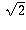can be represented as a solution to the quadratic equation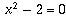, whose degree is 2. (Of course, it's also a solution to the cubic equation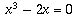whose degree is 3, not a power of 2; but that's because this equation can be factored, and after factoring out the redundant factor of x you are left with the quadratic, which is irreducible: it can't be factored any further and still have rational coefficients). If we take a square root a second time, getting the number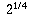, it's a root of the fourth-degree polynomial(which is irreducible). A number like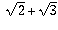, also obtained by twice taking a square root, is also the root of an irreducible fourth-degree polynomial: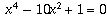. A number obtained by taking a square root three times, like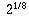, is typically the root of an irreducible 8th degree polynomial, like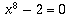.

This intuitive understanding is not always correct, though. For example,+, even though it is obtained using two square roots, is the same as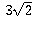, which involves only one square root. So the intuitive argument only serves to show you that the claims of the theorem are reasonable. Actually proving the theorem (and proving, not just that there's some irreducible polynomial equation for x whose degree is a power of 2, but that every irreducible polynomial equation for x also has that same degree) involves many advanced ideas.

Using the theorem, it is easy to prove the impossibility of the three constructions:

Doubling a cube is impossible because if you start with a cube of side length 1, you would need to cunstruct a cube whose side length is the cubed root of 2. But the the cubed root of 2 is a solution to the irreducible equation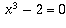whose degree, 3, is not a power of 2.

Squaring a circle is impossible because if you start with a circle of radius 1 you would need to construct a square whose side length is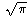. But this is a so-called "transcendental number": it is not the solution to any polynomial equation with rational coefficients, let alone one whose degree is a power of 2.

Trisecting an angle is impossible because if you start with an angle of 60 degrees (which is easily constructible), you would then need to be able to construct an angle of 20 degrees. This would be equivalent to constructing a point whose coordinates are the cosine and sine of 20 degrees. This is impossible because cos(20 degrees) is a solution to the irreducible polynomial equation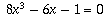whose degree, 3, is not a power of 2.

[ Submit Your Own Question ] [ Create a Discussion Topic ]

This part of the site maintained by (No Current Maintainers)
Last updated: April 19, 1999
Original Web Site Creator / Mathematical Content Developer: Philip Spencer
Current Network Coordinator and Contact Person: Joel Chan - mathnet@math.toronto.edu

Navigation Panel: